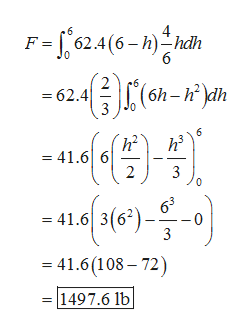# The trough in the figure below has width w=4, lengthL=20 ft and height h=6.If the trough is full of water, find the force of the water on a triangular end. (Use the density of water =62.4 lb/ft3.)Force = Don't forget to enter unitsFind the work to pump all of the water over the top of the trough.Work =

Question
86 views

The trough in the figure below has width w=4, lengthL=20 ft and height h=6.

If the trough is full of water, find the force of the water on a triangular end. (Use the density of water =62.4 lb/ft3.)
Force =
Don't forget to enter units

Find the work to pump all of the water over the top of the trough.
Work =

check_circle

Step 1

The base, height and length are 4ft, 6ft and 20ft, respectively.

Then the thickness of the triangle is (4/6) h Δh.

The distance that get after slicing the triangle is (6 – h).

Step 2

Then force ...help_outlineImage TranscriptioncloseF =62.4(6-h)^hdh (6h-yh =62.4 6 h3 = 41.6 6 2 3 63 41.6 3(62) 3 - 41.6(108-72) 1497.6 1b fullscreen

### Want to see the full answer?

See Solution

#### Want to see this answer and more?

Solutions are written by subject experts who are available 24/7. Questions are typically answered within 1 hour.*

See Solution
*Response times may vary by subject and question.
Tagged in

### Integration